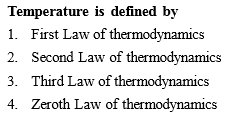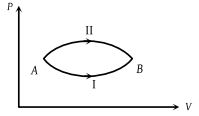Complete Question Bank + Test Series
Complete Question Bank

Difficulty Level:

At ${10}^{°}C$ the value of the density of a fixed mass of an ideal gas divided by its pressure is x. At ${110}^{°}C$ this ratio is

(a) x

(b)$\frac{383}{283}x$

(c)$\frac{10}{110}x$

(d) $\frac{283}{383}x$

Complete Question Bank + Test Series
Complete Question Bank

Difficulty Level:

Which of the following is not thermodynamical function ?

(1) Enthalpy

(2) Work done

(3) Gibb's energy

(4) Internal energy

Complete Question Bank + Test Series
Complete Question Bank

Difficulty Level:

Which of the following is not a thermodynamics co-ordinate ?

(1) P

(2) T

(3) V

(4) R

Complete Question Bank + Test Series
Complete Question Bank

Difficulty Level:

Which of the following statements is correct for any thermodynamic system ?

(1) The internal energy changes in all processes

(2) Internal energy and entropy are state functions

(3) The change in entropy can never be zero

(4) The work done in an adiabatic process is always zero

Complete Question Bank + Test Series
Complete Question Bank

Difficulty Level:

A monoatomic gas of n-moles is heated from temperature T1 to T2 under two different conditions (i) at constant volume and (ii) at constant pressure. The change in internal energy of the gas is

(1) More for (i)

(2) More for (ii)

(3) Same in both cases

(4) Independent of number of moles

Complete Question Bank + Test Series
Complete Question Bank

Difficulty Level:

In an isothermal change, an ideal gas obeys -

(1) Boyle's law

(2) Charle's law

(3) Gaylussac law

(4) None of the above

Complete Question Bank + Test Series
Complete Question Bank

Difficulty Level:

A gas mixture consists of 2 moles of oxygen and 4 moles argon at temperature T. Neglecting all vibrational modes, the total internal energy of the system is

(1) 4 RT

(2) 15 RT

(3) 9 RT

(4) 11 RT

Complete Question Bank + Test Series
Complete Question Bank

Difficulty Level:

Which one of the following gases possesses the largest internal energy ?

(1) 2 moles of helium occupying 1m3 at 300 K

(2) 56 kg of nitrogen at  Nm–2 and 300 K

(3) 8 grams of oxygen at 8 atm and 300 K

(4) 6 × 1026 molecules of argon occupying 40 m3 at 900 K

Complete Question Bank + Test Series
Complete Question Bank

Difficulty Level:

A system goes from A to B via two processes I and II as shown in figure. If ΔUI and ΔUII are the changes in internal energies in the processes I and II respectively, then(1) ΔUII > ΔUI

(2) ΔUII < ΔUI

(3) ΔUI = ΔUII

(4) Relation between ΔUI and ΔUII can not be determined